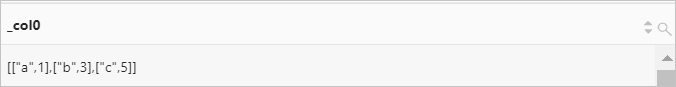Log Service allows you to define a lambda expression in an SQL analytic statement and pass the expression to a specified function. This topic describes the syntax of lambda expressions. This topic also provides examples on how to use the expressions.

## Syntax

You must use lambda expressions together with functions, such as filter function, reduce function, transform function, zip_with function, and map_filter function.

``parameter -> expression``
Parameter Description
parameter The identifier that is used to pass parameters.
expression The lambda expression, which can include most MySQL expressions. Examples:
``````x -> x + 1
(x, y) -> x + y
x -> regexp_like(x, 'a+')
x -> x / x
x -> if(x > 0, x, -x)
x -> coalesce(x, 0)
x -> cast(x AS JSON)
x -> x + try(1 / 0)``````

## Examples

### Example1: x -> x is not null

This lambda expression is used to return not-null elements in the [5, null, 7, null] array.

• Query statement
``* | SELECT filter(array[5, null, 7, null], x -> x is not null)``
• Query and analysis results### Example 2: 0, (s, x) -> s + x, s -> s

This lambda expression is used to return the sum of each element in the [5, 20, 50] array.

• Query statement
``* | SELECT reduce(array[5, 20, 50], 0, (s, x) -> s + x, s -> s)``
• Query and analysis results### Example 3: (k,v) -> v > 10

This lambda expression is used to create a map from two arrays. The values of keys in the map are greater than 10.

• Query statement
``* | SELECT map_filter(map(array['class01', 'class02', 'class03'], array[11, 10, 9]), (k,v) -> v > 10)``
• Query and analysis results### Example 4: (x, y) -> (y, x)

This lambda expression is used to transpose elements in two arrays and retrieve elements that are located by using the same index to form a new two-dimensional array.

• Query statement
``* | SELECT zip_with(array[1, 3, 5], array['a', 'b', 'c'], (x, y) -> (y, x))``
• Query and analysis results### Example 5: x -> coalesce(x, 0) + 1

This lambda expression is used to add 1 to each element in the [5, NULL, 6] array and return the result. The null element in the array is converted to 0 before it is added to 1.

• Query statement
``* | SELECT transform(array[5, NULL, 6], x -> coalesce(x, 0) + 1)``
• Query and analysis results``````* | SELECT filter(array[], x -> true)